# 15 – It’s Space Jim…

 < ρℝεν | ℂσητεητs | ℕεχτ >“The most powerful method of advance that can be suggested at present is to employ all the resources of pure mathematics in attempts to perfect and generalize the mathematical formalism that forms the existing basis of theoretical physics, and after each success in this direction, to try to interpret the new mathematical features in terms of physical entities.” – Paul Dirac

In the last Chapter we both formally defined the Special Unitary Group of degree 2, SU(2), and showed what a generic element of this looks like. The formal definition was as follows:

SU(2) is the set of 2 × 2 unitary matrices which also have a determinant of 1. We can write this as follows:Where w, x, y, z ∈ ℂ and det(A) means the determinant of matrix A.

And a generic member of SU(2) looks like this:Where a and b ∈ ℂ and |a|2 + |b|2 = 1.

If we want to move on to the next Special Unitary Group, i.e. SU(3), then the formal definition is easy to adapt from that of SU(2):

SU(3) is the set of 3 × 3 unitary matrices which also have a determinant of 1. We can write this as follows: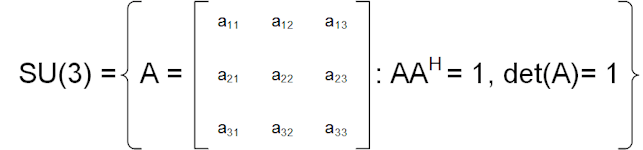Where aij ∈ ℂ and det(A) means the determinant of matrix A.

However determining what a generic member of SU(3) looks like will not be so straightforward and indeed not really possible in the economical manner we achieved for SU(2). Instead we are going to have to consider a set of eight 3 × 3 matrices which form a basis of the Lie algebra su(3) , which in turn generates the group SU(3).

Being fully aware that I have just introduced at least three new concepts in the space of a sentence, in this Chapter and the next I’m going to lay some of the groundwork necessary to make sense of the above. Later we will go on to look at how a similar approach can also generate SU(2) as well, before finally showing how to construct generic members of SU(3).

To start this journey, I’m going to go back to a rather fundamental concept, which we have touched on in passing a few times already, namely vectors.

The Red ArrowsWe first met vectors back in Chapter 5. Because at that point I was mostly focussed on explaining matrices, a brief definition of a vector was relegated to a footnote. However, in a box entitled What have Matrices ever done for us?, I did present the following diagram, which will now be of some help in more formally defining what a vector really is: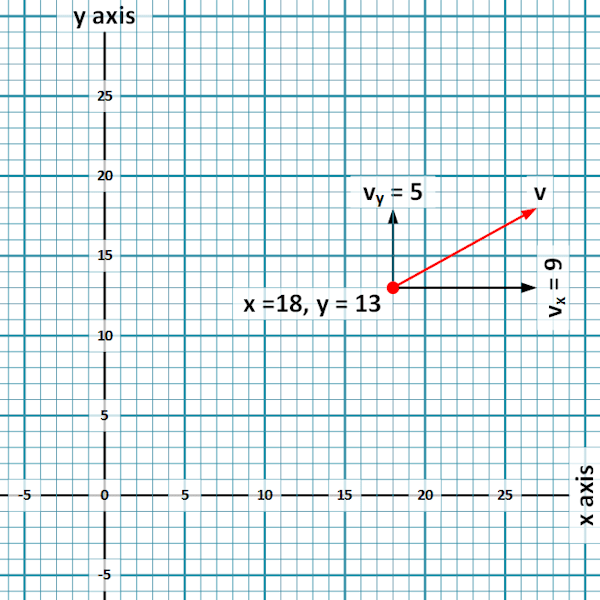To begin, I’ll stress that this is a visceral approach to vectors, one that is most frequently used to introduce schoolchildren to the subject. Mathematicians being Mathematicians, there is of course a more generalised / abstract definition, which we will get to soon. I do however think that this more physical starting point is probably a better place from which to establish an understanding of the subject.

The above image refers to a particle (the red dot) moving through a coordinate space (the “graph paper” background) in a particular direction (the direction of the red arrow) and with a particular velocity (the length of the red arrow). A physical definition of a vector is an “arrow” (in the sense of the arrow in the above diagram) which has a direction and a magnitude; in this case the arrow is labelled “v” .

Above it may be seen that our vector, v, can be specified wholly by the fact that it starts at point (18, 13) and ends at point (27, 18) in our coordinate system. These points encapsulate both the direction of the vector (draw a line between them)  and its magnitude (measure how long the line is). Here I have also decomposed the vector into components, each parallel to one of the axes of the coordinate system (vx parallel to the x-axis and vy parallel to the y-axis). This is a very common thing to do as certain calculations are made easier by such an approach. We shall come back to the idea of expressing vectors in terms of components later, but first I am more interested in the relationship between v, vx and vy.

Both vx and vy start at (18, 13), the same as v, so we can focus on their end points relative to this position, which are as follows:

vx = (+9, +0)

vy = (+0, +5)

Using this same approach relative to (18, 13) for v itself we see that:

v = (+9, +5)

and more particularly therefore that:

v = vx + vy

A moment’s thought will probably lead the reader to the conclusion that this final equality holds regardless of which starting point we choose.

While [this definition of] vectors are allowed to start anywhere, I’m going to avoid this complexity by considering vectors in a 2D coordinate space (again our graph paper, which has two axes and hence two dimensions) that all start from the origin, coordinates (0, 0) . We made an observation about adding the y-component of a vector, v, to the x-component to re-derive v itself. Let us now consider two vectors v and u both bound to the origin. What can we say about adding these?

Here a further geometric approach is going to help us visualise this operation:Here v’ is identical to v, save that its starting point has been moved to the end of u and symmetrically u’ is the same as u, save that its start point has been moved to the end of v. Here the geometric intuition is that v + u is the same as travelling along v and then, starting from where v finishes, travelling along u (which is equivalent to u’) .

Taking a more algebraic approach, given that both v and u start from (0, 0) we can define them wholly by their end points. Let’s generically say that these are (av, bv) and (au, bu) respectively. Then we define:

v + u = (av, bv) + (au, bu) = (av + au, bv + bu)

We can see this in the diagram above where travelling up and along v takes us av along the x-axis and travelling from there along u takes us a further au along the same axis; for a total distance travelled in the x direction of av + au. Considering the distance travelled parallel to the y-axis we similarly get bv + bu. Combining how far we have travelled with respect to both x- and y-axes gets us the overall coordinates of v + u being (av + au, bv + bu) as in the definition.

How do we Group Vectors?Having defined members of the set of bound vectors in a 2D Cartesian space, let’s call this V2D , and established how to add these (which is what we have just done), perhaps we could test a now well-known list of properties against (V2D,+) .

1. Closure

Well by our definition of addition:.

v + u = (av, bv) + (au, bu) = (av + au, bv + bu)

It is pretty clear that for any two vectors v and u ∈ V2D, v + u ∈ V2D, so we have closure.

2. Identity

If we recognise that the zero vector, (0, 0) (let’s denote this by 0) is clearly a member of V2D, then it follows that:

For all v ∈ V2D,

v + 0 = (av, bv) + (0, 0) = (av + 0, bv + 0)

= (av, bv)

So we have an identity element.

3. Inverses

If for a vector v = (av, bv) we consider the point (-av, -bv), then this also clearly defines a member of V2D, lets call it –v, and then we have:

v + –v = (av, bv) + (-av, -bv) = (av – av, bv – bv)

= (0, 0) = 0

So we have inverses.

4. Associativity

With the by now customary hand-waviness, as addition of vectors is driven by addition of their elements (the x-component and the y-component) and further as such addition is associative, so is addition of these types of vectors.

So, en passant as it were, we have found another Group. Indeed we may further observe that:

v + u = (av, bv) + (au, bu) = (av + au, bv + bu)

= (au + av, bu + bv)  = (au, bu) + (av, bv) = u + v

So the Group (V2D,+) is also Abelian.

So far everything I have done has applied to vectors starting from the origin of a 2D coordinate space. It must have occurred to readers that – even ignoring the mysterious generalisation of vectors I referred to above – we could think about vectors in a 3D coordinate space, or a 4D one, or indeed an n-dimensional coordinate space . Here a vector would be defined by respectively:

V3D: (a1, a2, a3) 

V4D: (a1, a2, a3, a4)

and

VnD: (a1, a2, … , an)

I won’t work these through long-hand, but – on the assumption that in each case we define vector addition by adding the relevant values for each coordinate axis together in an obvious extension of our 2D definition – it is probably fairly clear that all of these also form Groups under vector addition.

However my main motivation here (before returning to what this all means for Group Theory in Chapter 18), is to look at a new type of structure; one that includes the Group definitions we have got used to, but also some new properties. Let’s continue to use our 2D vectors as a vehicle for exploring these new properties before we start to get more general.

Tipping the ScalesIf we think back to Chapter 5 in which we introduced matrices, we looked at addition of these in precisely the same way that we have just looked at vector addition . We then went on to discuss a number of types of multiplication, the first of which was scalar multiplication, which for a 2 × 2 matrix A and a number λ is defined as follows: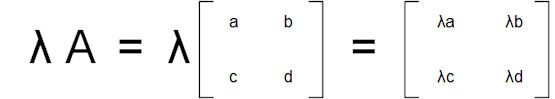Let’s now define scalar multiplication for members of V2D in a similar manner:

If v = (av, bv) ∈ V2D and λ is a number then:

λv = λ(av, bv) = (λav, λbv)

Again appealing to geometry, scalar multiplication is equivalent to stretching (or shrinking) a 2D vector by some factor as seen below.We might use this definition to note some properties of combining scalar multiplication and vector addition as follows:

λμ(av, bv) = (λμav, λμbv)

and also

λ(μav, μbv) = (λμav, λμbv)

so

(λμ)v = λ(μv)

So we things behave nicely when we combine multiplication of scalars with scalar multiplication.

1(av, bv) = (1av, 1bv)

= (av, bv)

So

1v = v

So there is also an Identity element with respect to scalar multiplication.

(λ + μ)(av, bv) = ((λ + μ)av, (λ + μ)bv)

= (λav + μav, λbv + μbv)

= (λav, λbv) + (μav, μbv)

So

(λ + μ)v = λv + μv

Which we call addition of scalars being distributive with respect to vector addition.

λ((av, bv) + (au, bu)) = λ(av + au, bv + bu)

= (λ(av + au), λ(bv + bu) = (λav + λau, λbv + λbu)

= (λav, λbv) + (λau, λbu)

= λ(av, bv) + λ(av, bv)

So

λ(v + u) = λv + λu

Which we call vector addition being distributive with respect to scalars.

These are certainly some interesting additional properties for (V2D,+vs)  to possess, but so what?

Well what I’m going to do here is to turn these observations on their head. What would we call a generic object (let’s call it V) with elements (let’s call them vectors) and two operators (let’s call them vector addition and scalar multiplication) that satisfy the rather long list of properties we have established above; both the four properties that make the object a Group under vector addition and the four new ones relating to scalar multiplication? The answer is a Vector Space and it is a definition of this concept that we have been stumbling towards so far in this Chapter and which we will explore more formally in the next.

Being Productive

Before moving on to looking at Vector Spaces more generally, I wanted to pause to briefly explore another couple of properties of the Cartesian vectors we have been considering. Both of these properties relate to multiplying vectors not by scalars but by other vectors. Mathematicians tend to like to refer to various types of multiplication as forming the product of two things and here I was going to look at two such products. These are both of intrinsic interest and will form the basis for more general properties that we will explore later in the book. The first is the dot product, also known as the inner product ; it will be leveraged in Chapter 22 when we define the concept of a Hilbert Space. The second is the cross product; it will come in useful in Chapters 18 when we introduce Lie Algebras.

1. Dot Product

Let’s work in a 2D coordinate space for now, we can generalise later. There are two equivalent ways of describing a dot product of two 2D vectors v = (vx, vy) and u = (ux, uy). The first is geometric and relates to noting that v and u will both have angles relative to the x-axis (θv and θu respectively) and an angle θ to each other, which may be derived from θv and θu. Diagrammatically we have: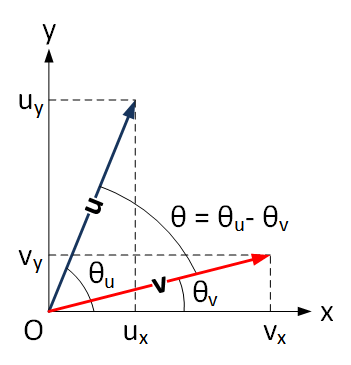The first definition of the dot product of v and u, written v.u for pretty obvious reasons, is:

v.u = |v||u| cos θ, where |x| denotes the size of vector x.

It should also be noted that if two vectors are at 90° to each other (orthogonal) then their dot product is zero (as cos 90° = 0). This geometric definition is pertinent in Physics where various properties of a system are represented by vectors and their dot product has an equally physical meaning .

The second definition is algebraic and in this example is given by:

v.u = vxux + vyuy

That is we multiply the values of each x-coordinate and those of each y-coordinate and sum.

The following box establishes the equivalence of these two definitions and may be skipped if the reader is already convinced that they are the same or (perhaps more likely) is ready to trust that this is the case.

 Looking at the diagram above, and recalling the basic trigonometry we employed in both Chapter 6 and Chapter 13, we can note the following: vx = |v| cos θv vy = |v| sin θv ux = |u| cos θu uy = |u| sin θu          (1) The same diagram tells us that θ = θu – θv. Noting this, and starting with our geometric definition, we can say that: v.u = |v||u| cos θ = |v||u| cos (θu – θv)          (2) But we can recall from Chapter 6 that: cos(A + B) = cos A cos B – sin A sin B So we can write (2) as: v.u = |v||u| (cos θu cos (-θv) – sin θu sin (-θv))          (3) And as cos (-A) = cos A and sin (-A) = -sin A, (3) can be written as: v.u = |v||u| (cos θu cos θv + sin θu sin θv) = |u| cos θu |v| cos θv + |u| sin θu |v| sin θv          (4) But using our definitions from (1) above we see that (4) is equivalent to: v.u = uxvx + uyvy Which is of course the algebraic definition. As we can derive one definition from the other (and vice versa as the operations above work both ways), they are equivalent.

Of course both definitions of dot product extend into higher dimensional spaces. It’s easier to do this for the algebraic formulation. Here if we have two n-dimensional vectors:

v = (v1, v2, v3, … , vn)

and

u = (u1, u2, u3, … , un)

then

v.u = v1u1 + v2u2 + … + vnun

Somewhat unaccountably I have got to Chapter 15 of this book without employing a summation symbol (a large sigma), but we would write this far more economically as:By employing approaches analogous to the one I have adopted above it can be shown that, most of the time, the two definitions remain equivalent in higher dimensions. However, the geometric definition is dependent on the concept of size of a vector, |v|, and in generalised Vector Spaces this concept is not always meaningful.

2. Cross Product

Our second product is called the cross product, denoted by v × u. Unlike the dot product above, the cross product has a rather limited existence, it is only defined in 3D space (so V3D(ℝ) using my shorthand), which is of course our familiar every day Euclidean space posessing up/down, back/front and left/right. Despite this rather narrow focus, the cross product is also very important in Physics (e.g. calculating angular momentum) and indeed Computer Graphics (manipulating polygons making up some virtual object). We will explore one specific Mathematical property of the cross product in Chapter 17.

While the dot product leads to a scalar result, the cross product generates a third vector. Again there are geometric and algebraic definitions. Let’s look at the geometric one first, which means another diagram.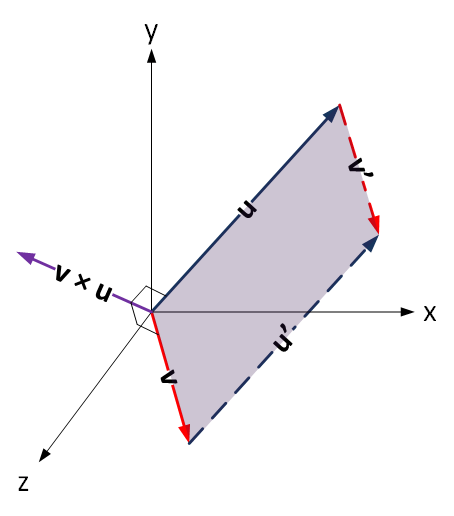It is always difficult to render vectors in 3D space, but I have attempted to do so in the above diagram. The x- and y-axes are as always left/right and up/down. The z-axis, which is perpendicular to both, is front/back and thus sticking out of the page. The two vectors I have drawn, v and u are meant to have components in all three axes and so also are poking out of the page.

The first thing to say is that any two vectors bound to the origin in a 3D space will lie in a plane – the transparent purple area shows part of this. Our cross product vector v × u is perpendicular to this plane (i.e. it is perpendicular to both v and u). There are clearly two choices of such vectors, one going down from the plane and one going up from it. The cross product is defined by a right hand rule of thumb. Orient the thumb, index and second fingers of your right hand so that they are all at 90° to each other, like the corner of a box. Turn your right hand so that your index finger points in the direction of the first vector in the cross product (v in this case) and your second finger points in the direction of the second vector (u in this case), then your thumb gives you the direction of v × u.

So we have a direction for the vector v × u, what about a magnitude? This is given by the area of the transparent purple parallelogram in the diagram.

That seems like a lot of calculation. However (and here we introduce the algebraic definition), if we have:

v = (vx, vy, vz)

and

u = (ux, uy, uz)

It can be shown  that:

v × u = (vyuz – vzuy, vzux – vxuz, vxuy – vyux)

So again we have an algebraic formulation of the cross product, one that will be easier to use when we look at this operator again in later Chapters.

Either definition of the dot product makes it pretty evident that it is commutative:

v.u = |v||u| cos θ = |u||v| cos θ = u.v

However if we consider the algebraic definition of the cross product we see that:

u × v = (uyvz – uzvy, vuvx – uxvz, uxvy – uyvx) =

-1 × (vyuz – vzuy, vzux – vxuz, vxuy – vyux) = – v × u

The property:

u × v = – v × u

is called anti-commutativity, a concept that applies to mathematical objects other that vectors, and something we will be meeting again in Chapter 17. In this case, the result makes physical sense as well. If you swap your index and second fingers over so that they each point to the other vector, you have to turn you hand over and your thumb moves through 180° to be pointing in the opposite direction.

So far we have been talking about coordinate spaces and vectors within these. We have rather airily ascribed the term Vector Space to these, but coordinate spaces are only one type of Vector Space. Vector Spaces are more general Mathematical objects and it is generalised Vector Spaces that is the subject of the next Chapter. So far we have been rather relaxed about what a Vector Space actually is. Mathematicians tend to abhor informality, so the next task is to be more specific about the rules for whether something is, or is not, a Vector Space.

 Concepts Introduced in this Chapter Vectors Viscerally, lines in a coordinate space which have both a magnitude and a direction; i.e. arrows of a certain length. More generally any Mathematical object which satisfies the same properties that the aforementioned arrows possess, but which may not share the same easily comprehended physical characteristics. Vector addition The combination of two vectors. Where these are arrow-like structures, equivalent to traversing the first vector and then the second starting from where the first one finished. In a Cartesian coordinate system, the same as adding the each of the coordinates of two vectors separately. Scalar multiplication of vectors Multiplying a vector by some number, commonly something like a Rational, Real or Complex Number. More generally by a member of a Field (see below). In a coordinate space, scalar multiplication is achieved by multiplying each coordinate of a vector by the scalar in turn. Dot product of vectors Generally multiplying each element of one vector by the corresponding element of another vector and then adding up the results. So if we have: v = (v1, v2, v3, … , vn) and u = (u1, u2, u3, … , un) thenCross product of vectors This is defined only for 3D space. For two vectors: v = (vx, vy, vz) and u = (ux, uy, uz) then v × u = (vyuz – vzuy, vzux – vxuz, vxuy – vyux) Anti-commutativity While in this Chapter we referenced this for vectors, for any binary operator, say ○, this is the property that: a ○ b = – b ○ a
 Groups Discovered in this Chapter (V2D,+) The Group of all vectors, bound to the origin in a 2D Cartesian coordinate space, under vector addition. (VnD,+) The Group of all vectors, bound to the origin in a n-dimensional Cartesian coordinate space, under vector addition.
 < ρℝεν | ℂσητεητs | ℕεχτ >

Chapter 15 – Notes

  In normal circumstances, Lie algebras are denoted by their names appearing in one or other variant of the Gothic Blackletter font, Fraktur. So this Lie Algebra should be written: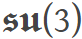While this works perfectly happily in PC browsers, it fails to render on most mobile browsers, so I have stuck to bold italics instead.  What we are considering here is more properly described as Cartesian Vectors (vectors in a Cartesian space) or sometimes geometric vectors. We will come on to more generalised vectors later  The direction is also defined by the start and end points. So a vector with a start point of (27, 18) and an end point of (18, 13) is different to v which has a start point of (18, 13) and and an end point of (27, 18). This vector with the opposite direction is actually –v.  What I am doing here is moving between free [Cartesian] vectors and bound [Cartesian] vectors, the latter all emanate from the origin of a given coordinate system.  Or, as addition is clearly commutative here, the same as travelling along u and then, starting from where u finishes, travelling along v (which is equivalent to v’).  This isn’t standard notation, just me looking for some shorthand to employ.  Sadly, there is no prize for guessing which.  As addition of numbers is commutative, 1 + 18.49 = 18.49 + 1.  Indeed we could also think about coordinate spaces other than Cartesian ones. There are non-Cartesian spaces which are still Euclidean (e.g. where axes are at some angle other than 90° to each other) and also non-Euclidean spaces, such as Riemannian manifolds.  Having used (av, bv) as our generic vector in 2D space, and (a1, a2, a3) as its equivalent in 3D space, it will be appreciated here that I am playing rather fast and loose with my subscripts; hopefully the meaning remains clear. At the end of the day bv and a2 are both meant to denote numbers and nothing else; precisely what type of numbers is something we will address soon.  Indeed I also suggested both that vectors could be viewed as n × 1 matrices, or that matrices could be viewed as collections of vectors.  Here I am using +v to denote vector addition and ×s to denote scalar multiplication; neither of these are standard terminology.  We actually made reference to this back in Chapter 5 when discussing different types of matrix multiplications. The Inner Product is actually a more generic version of the dot product, we will meet this in Chapter 17.  For example we might define vectors covering the force acting on a particle and its displacement resulting from this. The dot product of these two vectors is the work done.  Though, after the calculations in the previous box, I have no intention of doing so here.
 Text: © Peter James Thomas 2016-17. Images: © Peter James Thomas 2016-17, unless stated otherwise. Published under a Creative Commons Attribution 4.0 International License.